Test: Digital Voltmeter (DVM)

# Test: Digital Voltmeter (DVM) - Electrical Engineering (EE)

Test Description

## 10 Questions MCQ Test GATE Electrical Engineering (EE) 2024 Mock Test Series - Test: Digital Voltmeter (DVM)

Test: Digital Voltmeter (DVM) for Electrical Engineering (EE) 2023 is part of GATE Electrical Engineering (EE) 2024 Mock Test Series preparation. The Test: Digital Voltmeter (DVM) questions and answers have been prepared according to the Electrical Engineering (EE) exam syllabus.The Test: Digital Voltmeter (DVM) MCQs are made for Electrical Engineering (EE) 2023 Exam. Find important definitions, questions, notes, meanings, examples, exercises, MCQs and online tests for Test: Digital Voltmeter (DVM) below.
Solutions of Test: Digital Voltmeter (DVM) questions in English are available as part of our GATE Electrical Engineering (EE) 2024 Mock Test Series for Electrical Engineering (EE) & Test: Digital Voltmeter (DVM) solutions in Hindi for GATE Electrical Engineering (EE) 2024 Mock Test Series course. Download more important topics, notes, lectures and mock test series for Electrical Engineering (EE) Exam by signing up for free. Attempt Test: Digital Voltmeter (DVM) | 10 questions in 30 minutes | Mock test for Electrical Engineering (EE) preparation | Free important questions MCQ to study GATE Electrical Engineering (EE) 2024 Mock Test Series for Electrical Engineering (EE) Exam | Download free PDF with solutions
 1 Crore+ students have signed up on EduRev. Have you?
Test: Digital Voltmeter (DVM) - Question 1

### In a dual slope integrating type DVM, the accuracy of measured voltage ________ on the integrating time constant and ______ of frequency of oscillation.

Detailed Solution for Test: Digital Voltmeter (DVM) - Question 1

Dual slope integrating type is the popular method of Analog to Digital conversion. In dual slope method, the noise is averaged out by the positive and negative ramps using the process of integration.
The basic principle of this method is that the input signal is integrated for a fixed interval of time and then same integrator is used to integrate the reference voltage with reverse slope.
Hence the name is given to the technique is dual slope integration technique.
Dual slope integrating type ADC is the most accurate type of ADC because of  non-dependency on variation in components values caused by noise.
These reduce design complexity and improves measurement quality.
The accuracy of the measured voltage is inde­pendent of the integrator time constant and the accuracy of the method is also independent of the oscillator frequency.

Test: Digital Voltmeter (DVM) - Question 2

### What is the resolution of 4­-digit digital measuring instrument?

Detailed Solution for Test: Digital Voltmeter (DVM) - Question 2

Concept:

Resolution: It is the smallest increment an instrument can detect and display.

• The resolution for the 'N' bit digital voltmeter is given by: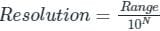• The range of an instrument is the sum of the smallest and the largest number it can display.

For 4­-digit, the smallest and the largest number that can be displayed is: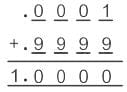Range = 1
Resolution = 1/104
Resolution = 10-4 = 0.0001
In percentage, the resolution is 0.01%

Test: Digital Voltmeter (DVM) - Question 3

### Which of the following is an advantage of the analog multimeter over the digital multimeter?

Detailed Solution for Test: Digital Voltmeter (DVM) - Question 3

Digital multimeters have the following advantages over analog multimeters:

• More accurate
• Reduced reading and interpolation errors
• Parallax error is eliminated
• Faster and do not require zero adjustment
• Smaller size therefore portable

However, analog meters have some advantages over digital multimeters, cheaper and less electric noise.

Test: Digital Voltmeter (DVM) - Question 4

In a digital voltmeter, during start of conversion, zero indication is displayed and is called auto zeroing. This is achieved by

Detailed Solution for Test: Digital Voltmeter (DVM) - Question 4

In a digital voltmeter, during start of conversion, zero indication is displayed. This is known as auto zeroing.
The circuit is used for this purpose is shown below.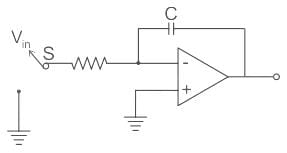It consists of an integrator. To achieve auto zeroing, the switch S is connected from Vin to the ground potential and the capacitor C of the dual-slope integrator discharges to the ground.

Test: Digital Voltmeter (DVM) - Question 5

A DVM has a 3½ digit display. The 1-volt range can read upon

Detailed Solution for Test: Digital Voltmeter (DVM) - Question 5

The resolution in a N bit DVM is given by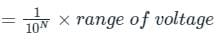Where N is the number of full digits.
In a DVM, a full digit counts 0 to 9 and half digit counts from 0 to 1.

Calculation:
The given DVM has 3 ½ digit display, i.e. it has 3 full digits and 1 half digit.
Resolution for the given DVM is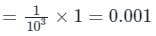Minimum count for the given DVM = 0.001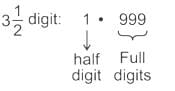Maximum count = 1.999 V

Test: Digital Voltmeter (DVM) - Question 6

In a digital multi-meter, AC voltage, DC current, AC current and resistance are first converted into equivalent ________ by a device and then measured with the help of a digital voltmeter.

Detailed Solution for Test: Digital Voltmeter (DVM) - Question 6

Digital Multimeter:

• A digital multimeter is an electronic instrument that can measure very precisely the dc and ac voltage, current (dc and ac), and resistance.
• All quantities other than dc voltage are first converted into an equivalent dc voltage by some device and then measured with the help of a digital voltmeter.
• Multimeters measure resistance by injecting a small current into the circuit and then measuring the voltage drop across those points in the circuit.
• The known current and the resulting voltage drop are then used to calculate the resistance using Ohm's Law.
Test: Digital Voltmeter (DVM) - Question 7

A reading of 100V on a digital multimeter ranges from 97V to 103V. Compute the accuracy.

Detailed Solution for Test: Digital Voltmeter (DVM) - Question 7

Digital multimeter:

• Digital multimeter present measurement data in a direct format that does not require calculating the exact value, and they are free from the parallax error of analog meters.
• Unlike meter movements in analog meters, digital multimeter displays have no moving parts, and they are free from wear and shock failures.
• Digital multimeters automatically detect polarity, show positive and negative values, have much better overload protection, and offer automatic as well as manual range selection options.

Calculation: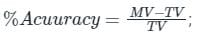Here, MV = Measured value
TV =  True value
Given, Lowest range of Measured Value(ML) = 97
Highest range of Measured Value(MH) = 103
True Value = 100
therefore,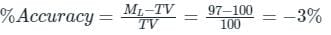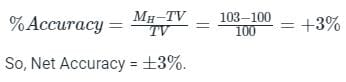Test: Digital Voltmeter (DVM) - Question 8

A voltmeter connected across 15 kΩ resistor reads 10 V in the circuit given. Voltmeter is rated at 500 Ω / volt and has a full- scale reading of 20 V. The supply voltage is: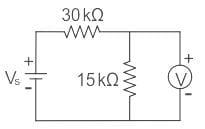Detailed Solution for Test: Digital Voltmeter (DVM) - Question 8

Concept:

The voltmeter sensitivity (Sv) is determined by dividing the sum of the resistance of the meter (Rm)

Mathematically, sensitivity is expressed as: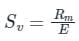E = Rated Voltage or Full scale Reading
Voltmeter sensitivity is expressed in Ohm/Volt
Rm = Sv × E

Calculation:
Sensitivity of voltmeter = 500 Ω/V
Internal resistance of voltmeter = 500 ×
20 = 10 kΩ
Effective resistance across A – B is
= 10 || 15 = 6 kΩ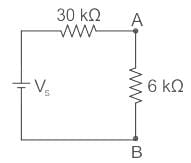The voltmeter reading is 10 V
⇒ VAB = 10 V
By voltage division,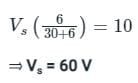Test: Digital Voltmeter (DVM) - Question 9

Multiplication features are incorporated in an ohmmeter to enable the meter to ________.

Detailed Solution for Test: Digital Voltmeter (DVM) - Question 9

To enable the ohmmeter to indicate any value being measured, with the least error, scale multiplication features are used in most ohmmeters.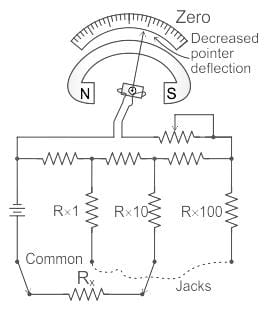• A typical meter will have four test lead jacks: COMMON, R X 1, R X 10, and R X 100
• The jack marked COMMON is connected internally through the battery to one side of the moving coil of the ohmmeter
• The jacks marked R X 1, R X 10, and R X 100 are connected to three different size resistors located within the ohmmeter
Test: Digital Voltmeter (DVM) - Question 10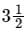digit voltmeter has the resolution of

Detailed Solution for Test: Digital Voltmeter (DVM) - Question 10

Concept:
The resolution (R) in an N bit DVM is given by: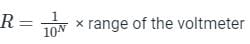Where N is the number of full digits.
In a DVM, a full digit counts 0 to 9 and a half digit counts from 0 to 1.
For N and half DVM resolution (R) is given by: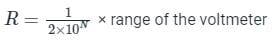Calculation:
Given that,
N = 3(1/2)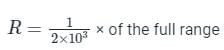%R = (1/2000) × 100 × of full range
%R = 0.05 % of full range

## GATE Electrical Engineering (EE) 2024 Mock Test Series

23 docs|285 tests
Information about Test: Digital Voltmeter (DVM) Page
In this test you can find the Exam questions for Test: Digital Voltmeter (DVM) solved & explained in the simplest way possible. Besides giving Questions and answers for Test: Digital Voltmeter (DVM), EduRev gives you an ample number of Online tests for practice

## GATE Electrical Engineering (EE) 2024 Mock Test Series

23 docs|285 tests